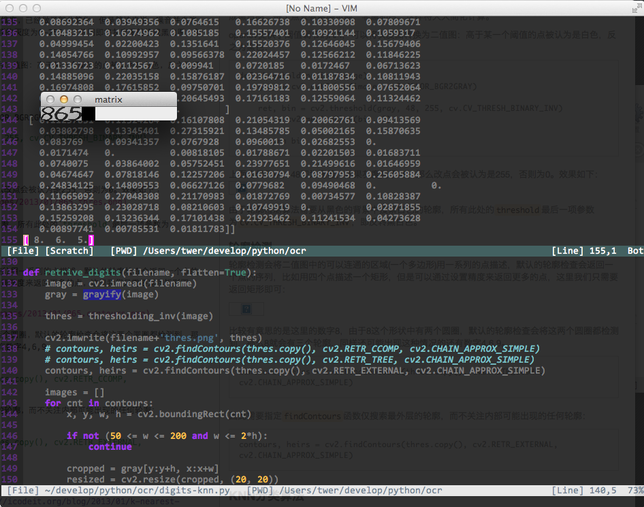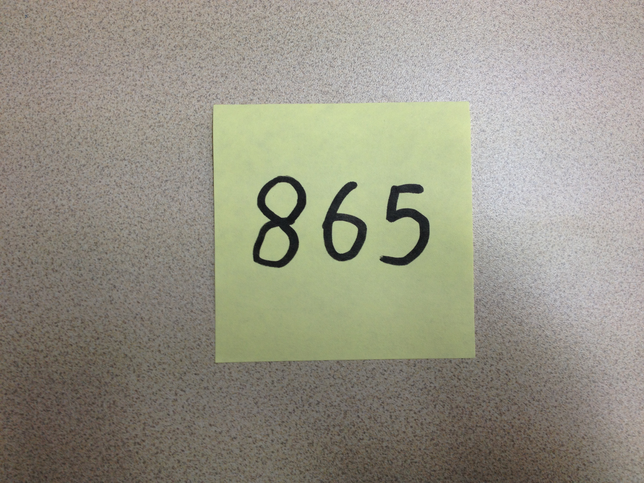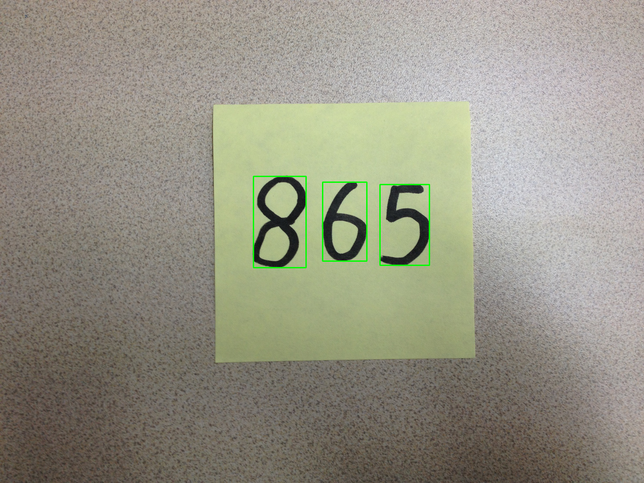``````1. ROI抽取即将感兴趣的区域从原始图像中分离初来，这个步骤包括二值化，噪点的消除等
2. 识别即通过一些分类器将第一步中的结果进行分类，事实上属于机器学习的一个典型应用
``````

###图像预处理####灰度图 通常的彩色图形由3个(RGB)或者4个(RGBA)通道组成，在计算机看来，一个彩色的图片是由3/4个矩阵组成，每个矩阵中包含若干个点(比如1024x768)，如果每个通道都参与运算的话，会引入太多的计算量，因此通常的做法是将彩色图像转换为灰度图，在opencv中，这一步非常容易：

``````def grayify(image):
return cv2.cvtColor(image, cv2.COLOR_BGR2GRAY)

``````

####二值图 灰度图较之原始图片，将三个维度的矩阵变成了一个维度，已经做了部分简化，但是算法来说，噪音并未消除，灰度图中，每个点仍然有8位来表示，每个点可能的灰度为0-255，二值图即将灰度图转换成黑白图，每个点只有两种可能：非黑即白，这样将大大简化计算。

opencv提供了阈值调节的API，可以将灰度图转换为二值图：高于某一个阈值的点被认为是白色，反之为黑色：

``````def thresholding_inv(image):
gray = cv2.cvtColor(image, cv2.COLOR_BGR2GRAY)

ret, bin = cv2.threshold(gray, 48, 255, cv.CV_THRESH_BINARY_INV)
bin = cv2.medianBlur(bin, 3)

return bin
``````

####轮廓检测 轮廓检测会将二值图中的可以连通的区域(一个多边形)用一系列的点描述，默认的轮廓检查会返回一个点的序列，比如用四个点描述一个矩形，但是可以通过设置精度来返回更多的点，这里我们只需要返回矩形即可：``````contours, heirs = cv2.findContours(thres.copy(), \
cv2.RETR_CCOMP, cv2.CHAIN_APPROX_SIMPLE)
``````

``````contours, heirs = cv2.findContours(thres.copy(), \
cv2.RETR_EXTERNAL, cv2.CHAIN_APPROX_SIMPLE)
``````

###KNN分类算法 KNN算法的原理可以参看之前的一篇文章。这里的实现主要参考了opencv的示例程序:

``````class KNearest(StatModel):
def __init__(self, k = 3):
self.k = k
self.model = cv2.KNearest()

def train(self, samples, responses):
self.model = cv2.KNearest()
self.model.train(samples, responses)

def predict(self, samples):
retval, results, neigh_resp, dists = \
self.model.find_nearest(samples, self.k)
return results.ravel()
``````

####数字的顺序 另外一个有意思的事情是轮廓检测的时候，算法并不一定按照从左到右，从上到下的方向进行，我开始只是简单的按照迭代的顺序将结果插入到一个list中，但是这样list中的结果是乱的，下午得到了team中有图像处理背景的杨眉同学的支持：搜索到轮廓的时候，将此时的position信息与轮廓一起记录下来，然后在搜索完成之后，将整个列表按照x坐标排序(卡上的数字是按照从左向右书写)：

``````class PosImage(object):
def __init__(self, pos, image):
self.pos = pos
self.image = image

def get_position(self):
return self.pos

def get_image(self):
return self.image

``````

``````    cropped = gray[y:y+h, x:x+w]
resized = cv2.resize(cropped, (20, 20))
cv2.rectangle(image, (x, y), (x+w, y+h), (0, 255, 0), 3)
pos_image = PosImage((x, y), resized)
images.append(pos_image)
``````

``````def rearrange(images):
return sorted(images, cmp=lambda x, y:
cmp(x.get_position(), y.get_position()))

``````